# I.E. Irodov Solutions on Dynamics OF A Solid Body

The solutions of the Problems In General Physics I.E. Irodov - Dynamics Of A Solid Body are given on this page. Dynamics Of A Solid Body is an important topic in the Physical Fundamentals of Mechanics. The important topics include the equation of dynamics of a solid body rotating about a stationary axis, Steiner's theorem, Kinetic energy of a solid body rotating about a stationary axis, Kinetic energy of a solid body in-plane motion, Relationship between the angular velocity of gyroscope precession, its angular momentum and the moment of the external forces, etc.

Practising these solutions will help students to boost their confidence and face the exam in a stress-free manner.

### I.E. Irodov Solutions on Dynamics OF A Solid Body

1. A thin uniform rod AB of mass m = 1.0 kg moves translationally with acceleration w = 2.0 m/s2 due to two antiparallel forces F1 and F2 (Fig. 1.52). The distance between the points at which these forces are applied is equal to a = 20 cm. Besides, it is known that F2 = 5.0 N. Find the length of the rod.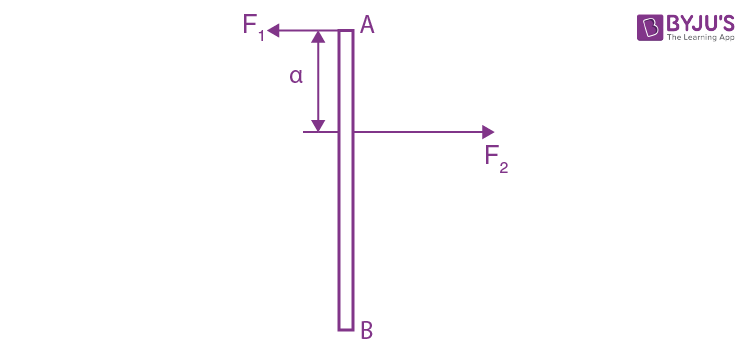Solution:

1. Since, motion of the rod is purely translational, net torque about the C.M of the rod should be equal to zero.

Thus F1l/2 = F2(l/2 - a)

Or F1/F2 1 - a/(l/2) (1)

For the translational motion of rod

F2 - F1 = mwc

Or 1 - F1/F2 = mwc/F2 (2)

From (1) and (2)

a/(l/2) = mwc/F2

Or l = 2aF2/mwc = 1m

2. A force F = Ai + Bj is applied to a point whose radius vector relative to the origin of coordinates O is equal to r = ai + bj, where a, b, A, B are constants, and i, j are the unit vectors of the x and y axes. Find the moment N and the arm l of the force F relative to the point O.

Solution:

1. Sought moment $\vec{N}=\vec{r}\times \vec{F}$

= $(a\vec{i}+b\vec{j})\times (A\vec{i}+B\vec{j})$

= $(aB\vec{k}+Ab(-\vec{k}))$

= $(aB-Ab)\vec{k}$

And arm of the force l = N/F = (aB - Ab)/√(A2 + B2)

3. A force F1 = Aj is applied to a point whose radius vector ri = ai, while a force F2 = Bi is applied to the point whose radius vector r2 = bj . Both radius vectors are determined relative to the origin of coordinates O, i, and j are the unit vectors of the x and y axes, a, b, A, B are constants. Find the arm l of the resultant force relative to the point O.

Solution:

1. Relative to point O, the net moment of force

$\vec{N}=\vec{r}_{1}\times \vec{F}_{1}+\vec{r}_{2}\times \vec{F}_{2}$

= $(a\vec{i}\times A\vec{j} )+(B\vec{j}\times b\vec{i} )$

= $(ab-AB)\vec{k}$

Resultant of the external force

$\vec{F} = \vec{F}_{1}+\vec{F}_{2}=A\vec{j}+B\vec{i}$

As $\vec{N} .\vec{F} =0$

as $\vec{N}$ perpendicular to $\vec{F}$ so the sought arm l of the force $\vec{F}$

l = N/F = (ab - AB)/√(A2 + B2)

4. Three forces are applied to a square plate as shown in Fig. 1.53. Find the modulus, direction, and the point of application of the resultant force, if this point is taken on the side BC.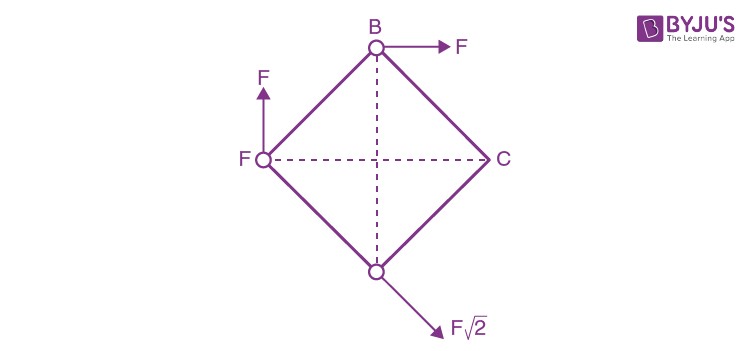Solution:

1. For coplanar forces, about any point in the same plane

$\sum \vec{r}_{i}\times \vec{F}_{i}=\vec{r}\times \vec{F}_{net}$

Where $\vec{F}_{net}=\sum \vec{F}_{i}$ = resultant force

Or $\vec{N}_{net}=\vec{r} \times \vec{F}_{net}$

Thus length of the arm, l = Nnet/Fnet

Here obviously $\left | \vec{F}_{net} \right |=2F$ and it is directed toward right along AC. Take the origin at C. Then about C,

$\vec{N}=\sqrt{2}aF+\frac{a}{\sqrt{2}}F-\sqrt{2}aF$ directed normally into the plane of figure. Here a = side of the square.

Thus $\vec{N}=F\frac{a}{\sqrt{2}}$ directed into the plane of the figure.

Hence l = F(a/√2)/2F = a/2√2 = (a/2)sin 450

Thus the point of application of force is at the midpoint of the side BC.

5. Find the moment of inertia

1. (a) of a thin uniform rod relative to the axis which is perpendicular to the rod and passes through its end, if the mass of the rod is m and its length l;

2. (b) of a thin uniform rectangular plate relative to the axis passing perpendicular to the plane of the plate through one of its vertices, if the sides of the plate are equal to a and b, and its mass is m.

Solution:

1. (a)Consider a strip of length dx at a perpendicular distance x from the axis about which we have to find the moment of inertia of the rod. The elemental mass of the rod equals

dm = (m/l)dx

Moment of inertia of this element about the axis

dI = dmx2 = (m/l)dx. x2

Thus, the moment of inertia of the rod, as a whole about the given axis

I = ∫0l (m/l)x2 dx

= ml2/3

(b) Let us imagine the plane of the plate as xy plane taking the origin at the intersection point of the sides of the plate (Fig.).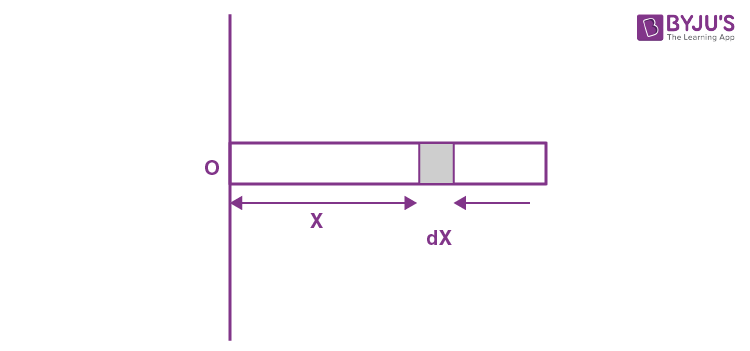Obviously Ix = ∫ dm y2

= $\int_{0}^{a}(\frac{m}{ab}b\: dy)y^{2}$

= ma2/3

Similarly Iy = mb2/3

Hence from the perpendicular axis theorem

Iz= Ix + Iy

= (m/3)(a2 + b2), which is the sought moment of inertia.

6. Calculate the moment of inertia

1. (a) of a copper uniform disc relative to the symmetry axis perpendicular to the plane of the disc, if its thickness is equal to b = 2.0 mm and its radius to R = 100

2. (b) of a uniform solid cone relative to its symmetry axis, if the mass of the cone is equal to m and the radius of its base to R.

Solution:

1. Consider an elementary disc of thickness dx. Moment of inertia of this element about the z-axis, passing through its C.M.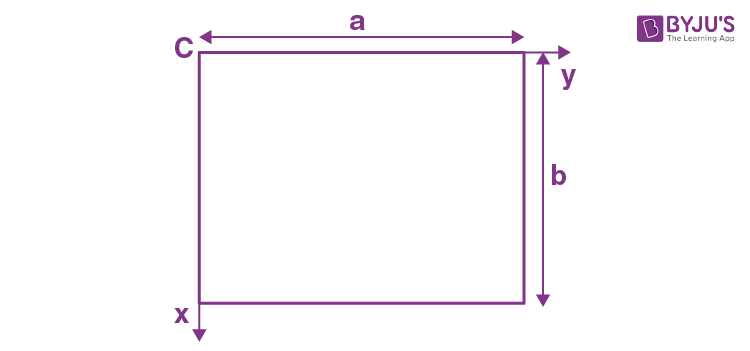dIz = (dm)R2/2 = ρS dx R2/2

where ρ = density of the material of the plate and S = area of cross-section of the plate. Thus the sought moment of inertia

$I_{z}=\frac{\rho SR^{2}}{2}\int_{0}^{b}dx$

= R2ρSb/2

= πρbR4/2 (as S = πR2)

putting all the values we get, lz = 2.gm.m2

(b) Consider an element disc of radius r and thickness dx at a distance x from the point O. Then r = x tan α and volume of the disc = πx2 tan2 α dx

Hence, its mass dm = πx2 tan α dx.ρ (where ρ = density of the cone = m/(⅓)πR2h)

Moment of inertia of this element, about the axis OA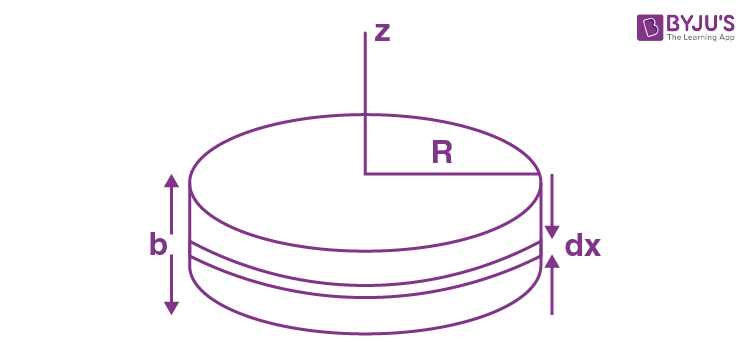dI = dm(r2/2)

= πx2 tan2 α dx(x2 tan2 α)/2

= (πρ/2)x4 tan4 α dx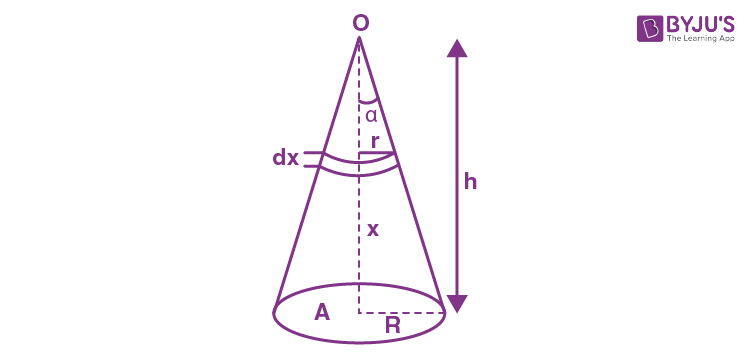Thus the sought moment of inertia I = (πρ/2) tan4 α ∫0h x4 dx

= πρR4h5/10h4 (as tan α = R/h)

Hence I = 3mR2/10 (putting ρ = 3m/πR2h)

7. Demonstrate that in the case of a thin plate of arbitrary shape there is the following relationship between the moments of inertia: l1+ I2 = l3, where subindices 1, 2, and 3 define three mutually perpendicular axes passing through one point, with axes 1 and 2 lying in the plane of the plate. Using this relationship, find the moment of inertia of a thin uniform round disc of radius R and mass m relative to the axis coinciding with one of its diameters.

Solution:

1. (a) Let us consider a lamina of an arbitrary shape and indicate by 1,2 and 3, three axes coinciding with x, y, and z axes and the plane of lamina as x-y plane.

Now, moment of inertia of a point mass about x-axis, dIx= dm y2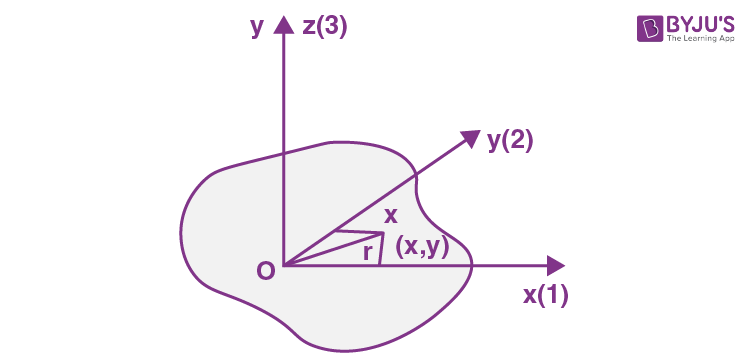Similarly, Iy = ∫dm x2

And Iz= ∫dm r2

= ∫dm (x2 + y2) as r = √(x2 + y2)

Thus Iz = Ix + Iy or I3 = I2 + I1

(b) Let us take the plane of the disc as x-y plane and origin to the centre of the disc (Fig.) From the symmetry Ix = Iy. Let us consider a ring element of radius r and thickness dr, then the moment of inertia of the ring element about the y axis.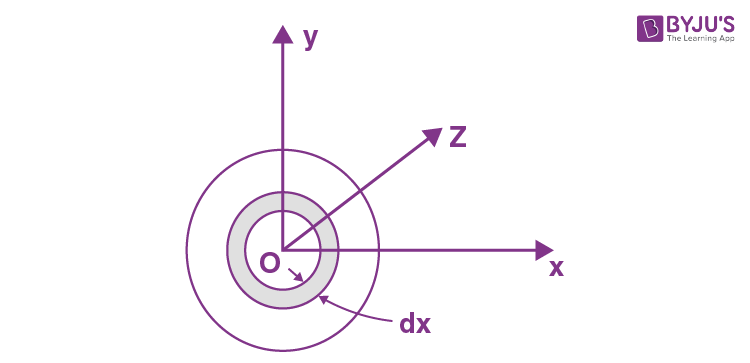dIz = dmr2 = (m/πR2)(2πr dr)r2

Thus the moment of inertia of the disc about z-axis.

Iz = $\frac{2m}{R^{2}}\int_{0}^{R}r^{3}dr=\frac{mR^{2}}{2}$

But we have Iz = Ix + Iy = 2Ix

Thus Ix = Iz/2 = mR2/4

8. A uniform disc of radius R = 20 cm has a round cut as shown in Fig. 1.54. The mass of the remaining (shaded) portion of the disc equals m = 7.3 kg. Find the moment of inertia of such a disc relative to the axis passing through its centre of inertia and perpendicular to the plane of the disc.Solution:

1. For simplicity let us use a mathematical trick. We consider the portion of the given disc as the superposition of two complete discs (without holes), one of positive density and radius R and the other of negative density but of the same magnitude and radius R/2.

As (area) α (mass), the respective masses of the considered discs are (4m/3) and (-m/3) respectively, and these masses can be imagined to be situated at their respective centers (C.M). Let us take point O as origin and point x-axis towards right. Obviously, the C.M. of the shaded position of the given shape lies on the x-axis. Hence the C.M. (C) of the shaded portion is given by

xc = ((-m/3)(-R/2( + (4m/3)0)/((-m/3) + 4m/3)

= R/6

Thus C.M. of the shape is at a distance R/6 from point O toward x-axis.

Using parallel axis theorem and bearing in mind that the moment of inertia of a complete homogeneous disc of radius m0 and radius r0 equals ½ m0r02. The moment of inertia of the small disc of mass (-m/3) and radius R/2 about the axis passing through point C and perpendicular to the plane of the disc

I2C = ½ (-m/3)(R/2)2 + (-m/3)(R/2 + R/6)2

= -mR2/24 - (4/27)mR2

Similarly I1C = ½ (4m/3)R2 + (4m/3)(R/6)2

= ⅔ mR2 + mR2/27

Thus the sought moment of inertia,

IC = I1C + I2C = (15/24)mR2 - (3/27)mR2 = (37/72)mR2

9. Using the formula for the moment of inertia of a uniform sphere, find the moment of inertia of a thin spherical layer of mass m and radius R relative to the axis passing through its centre.

Solution:

1. Moment of inertia of the shaded portion, about the axis passing through its centre,

I = (⅖)(4/3)πR3ρR2 - ⅖ (4/3) πr3ρr3

= ⅖ (4/3)πρ(R5 - r5)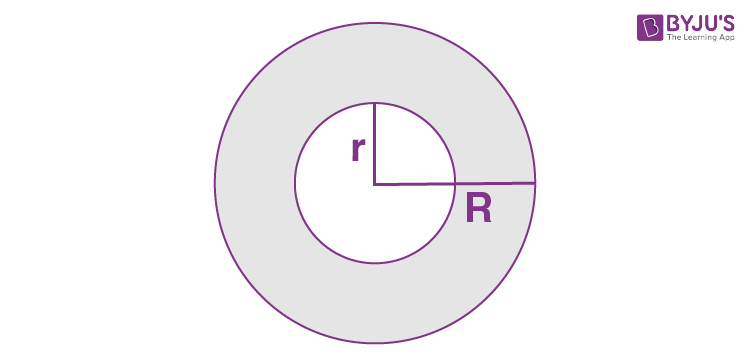Now, if R = r + dr, the shaded portion becomes a shell, which is the required shape to calculate the moment of inertia.

Now, I = ⅖ - (4/3) πρ( r + dr)5 - r5)

= ⅖ (4/3)πρ(r5 + 5r4dr + ….-r5)

Neglecting higher terms

= ⅔ (4πr2 dr ρ)r2

= ⅔ mr2

10. A light thread with a body of mass m tied to its end is wound on a uniform solid cylinder of mass M and radius R (Fig. 1.55). At a moment t = 0 the system is set in motion. Assuming the friction in the axle of the cylinder to be negligible, find the time dependence of

1. (a) the angular velocity of the cylinder;

2. (b) the kinetic energy of the whole system.

Solution:

1. (a) Net force which is effective on the system (cylinder M + body m) is the weight of the body m in a uniform gravitational field, which is a constant. Thus the initial acceleration of the body m is also constant.

From the conservation of mechanical energy of the said system in the uniform field of gravity at time t = ∆t: ∆T + ∆U = 0

Or ½ mv2 + ½ (MR2/2)ω2 - mg∆h = 0

Or ¼ (2m + M)v2 - mg∆h = 0 [as v = ωR at all times] (1)

But v2 = 2w∆h

Hence using it in Eq. (1), we get

¼ (2m + M)2w∆h - mg ∆h = 0 or w = 2mg/(2m + M)

From the kinematical relationship, β = w/R = 2mg/(2m + M)R

Thus the sought angular velocity of the cylinder ω(t) = βt = 2mgt/(2m + M)R = gt/(1 + M/2m)R

(b) sought kinetic energy

T(t) = ½ mv2 + ½ (MI2/2) ω2

= ¼ (2m + M)R2ω2

11. The ends of thin threads tightly wound on the axle of radius r of the Maxwell disc are attached to a horizontal bar. When the disc unwinds, the bar is raised to keep the disc at the same height. The mass of the disc with the axle is equal to m, the moment of inertia of the arrangement relative to its axis is I. Find the tension of each thread and the acceleration of the bar.

Solution:

1. For equilibrium of the disc and axle

2T = mg or T = mg/2

As the disc unwinds, it has an angular acceleration β given by

Iβ = 2Tr or β = 2Tr/I = mgr/I

The corresponding linear acceleration is

rβ = w = mgr2/I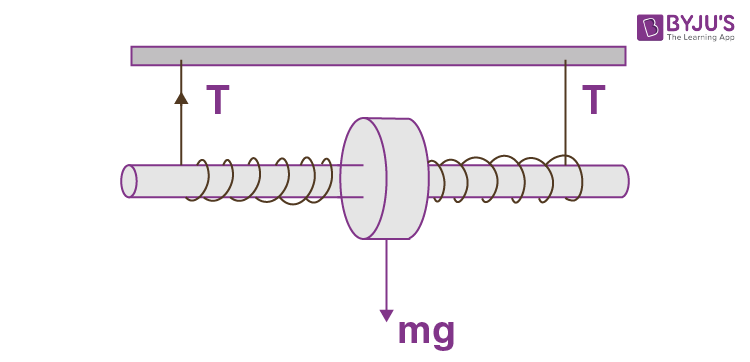Since the disc remains stationary under the combined action of this acceleration and the acceleration (-w) of the bar which is transmitted to the axle, we must have

w = mgr2/I

12. A thin horizontal uniform rod AB of mass m and length l can rotate freely about a vertical axis passing through its end A. At a certain moment the end B starts experiencing a constant force F which is always perpendicular to the original position of the stationary rod and directed in a horizontal plane. Find the angular velocity of the rod as a function of its rotation angle ϕ counted relative to the initial position.

Solution:

1. Let the rod be deviated through an angle ϕ’ from its initial position at an arbitrary instant of time, measured relative to the initial position in the positive direction. From the equation of the increment of the mechanical energy of the system

∆T = Aext

Or ½ Iω2 = ∫Nz

½ MI2ω2/3 = $\int_{0}^{\phi }Fl\cos \phi \: d\phi = Fl\sin \phi$

Thus ω = √(6F sin ϕ/Ml)

13. In the arrangement shown in Fig. 1.56 the mass of the uniform solid cylinder of radius R is equal to m and the masses of two bodies are equal to m1 and m2. The thread slipping and the friction in the axle of the cylinder are supposed to be absent. Find the angular acceleration of the cylinder and the ratio of tensions T1/T2 of the vertical sections of the thread in the process of motion.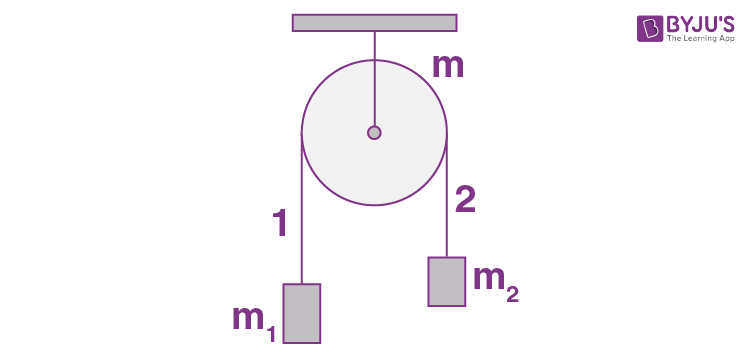Solution:

1. First of all, let us sketch free body diagram of each body. Since the cylinder is rotating and massive, the tension will be different in both the sections of threads. From Newton’s law in projection form for the bodies m1 and m2 and noting that w1 = w2 = w = βR, (as no thread slipping), we have (m1>m2).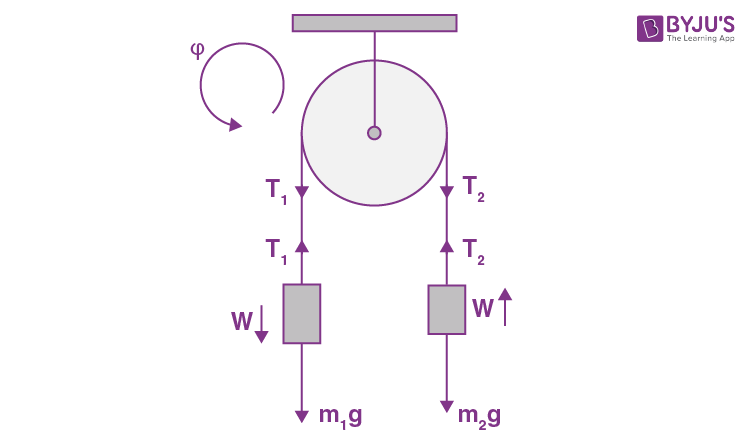m1g - T1 = m1w = m1βR

And T2 - m2g = m2w (1)

Now from the equation of rotational dynamics of a solid about stationary axis of rotation,

i.e. Nz= Iβz , for the cylinder.

Or (T1 - T2)R = Iβ = mR2β/2 (2)

Simultaneous solution of the above equation yields:

β = (m1 - m2)g/R(m1 + m2 + m/2) and T1/T2 = m1(m+4m2)/m2(m+4m1)

14. In the system shown in Fig. 1.57 the masses of the bodies are known to be m1 and m2 , the coefficient of friction between the body m1 and the horizontal plane is equal to k, and a pulley of mass m is assumed to be a uniform disc. The thread does not slip over the pulley. At the moment t = 0 the body m2 starts descending. Assuming the mass of the thread and the friction in the axle of the pulley to be negligible, find the work performed by the friction forces acting on the body m1 over the first t seconds after the beginning of motion.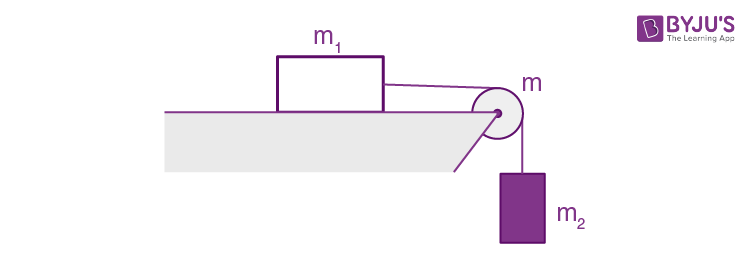Solution:

1. As the system (m + m1 + m2) is under constant forces,the acceleration of body m1 and m2 is constant. In addition to it the velocities and accelerations of bodies m1 and m2 are equal in magnitude (say v and w) because the length of the thread is constant. From the equation of increment of mechanical energy i.e. ∆T + ∆U = Afr, at time t when block m1 is distance h below from initial position corresponding to t = 0.

½ (m1 + m2)v2 + ½ (mR2/2)v2/R2 - m2gh = -km1gh (1)

(as angular velocity ω = v/R for no slipping of thread.)

But v2 = 2wh

So using it in (1), we get

w = 2(m2 - km1)g/(m + 2(m1 + m2)) (2)

Thus the work done by the friction force on m1

Afr = -km1gh = -km1g(½ wt2)

= -km1(m1- km1)g2t2/(m + 2(m1 + m2)) (using 2)

15. A uniform cylinder of radius R is spinned about its axis to the angular velocity ω0 and then placed into a corner (Fig. 1.58). The coefficient of friction between the corner walls and the cylinder is equal to k. How many turns will the cylinder accomplish before it stops?Solution:

1. In the problem, the rigid body is in translation equilibrium but there is an angular retardation. We first sketch the free body diagram of the cylinder.Obviously the friction forces, acting on the cylinder, are kinetic. From the condition of translational equilibrium for the cylinder,

mg = N1 + kN2;

N2 = kN1

Hence N1 = mg/(1 + k2)

N2 = kmg/(1 + k2)

For pure rotation of the cylinder about its rotation axis,

Nz = Iβz

Or, -kN1R - kN2R = mR2βz/2

Or, -kmgR(1+k)/(1+k2) = mR2βz/2

Or, βz = -2k(1+k)g/(1+k2)R

Now, from the kinematical equation,

ω2 = ω02 + 2βz∆ϕ we have

∆ϕ = ω02(1+k2)R/4k(1+k)g, because ω= 0

Hence the sought number of turns,

n = ∆ϕ/2π = ω02(1+k2)R/8πk(1+k)g

16. A uniform disc of radius R is spinned to the angular velocity ω and then carefully placed on a horizontal surface. How long will the disc be rotating on the surface if the friction coefficient is equal to k? The pressure exerted by the disc on the surface can be regarded as uniform.

Solution:

1. It is the moment of friction force which brings the disc to rest. The force of friction is applied to each section of the disc, and since these sections lie at different distances from the axis, the moments of the forces of friction differ from section to section.

To find Nz, where z is the axis of rotation of the disc let us partition the disc into thin rings (Fig.).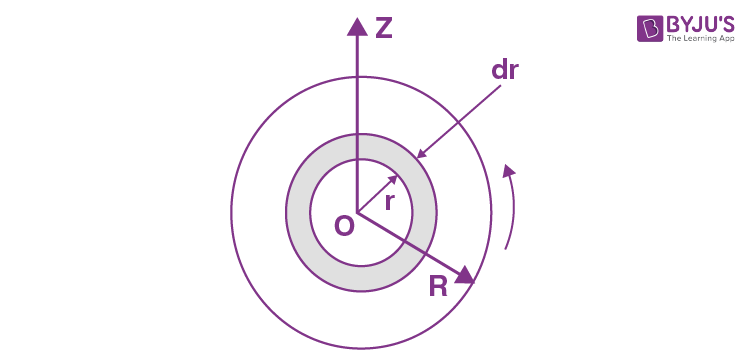The force of friction acting on the considered element.

dfr = k(2πr dr σ)g (where σ is the density of the disc)

The moment of this force of friction is

dNz = -r dfr = -2πkσ gr2 dr

Integrating w.r.t.r from zero to R, we get

Nz= -2πkσg∫0R r2 dr = -⅔ πkσgR3

For the rotation of the disc about the stationary axis z, from the equation Nz = Iβz

-⅔ πkσgR3 = (πR2σ)R2βz/2 or βz = -4kg/3R

Thus from the angular kinematical equation

ωz = ω0z + βzt

0 = ω0z + (-4kg/3R)t

Or t = 3R ω0/4kg

17. A flywheel with the initial angular velocity ω0 decelerates due to the forces whose moment relative to the axis is proportional to the square root of its angular velocity. Find the mean angular velocity of the flywheel averaged over the total deceleration time.

Solution:

1. According to the question

Idω/dt = -k√ω or I = dω/√ω = -k dt

Integrating, √ω = -kt/2I + √ω0

Or, ω = k2t2/4I2 - √ω0kt/I + ω0 (Noting that at t = 0, ω = ω0)

Let the flywheel stops at t = t0 then from Eq.(1) t0 = 2I √ω0/k

Hence sought average angular velocity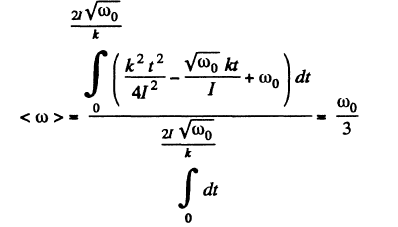18. A uniform cylinder of radius R and mass M can rotate freely about a stationary horizontal axis O (Fig. 1.59). A thin cord of length l and mass m is wound on the cylinder in a single layer. Find the angular acceleration of the cylinder as a function of the length x of the hanging part of the cord. The wound part of the cord is supposed to have its centre of gravity on the cylinder axis.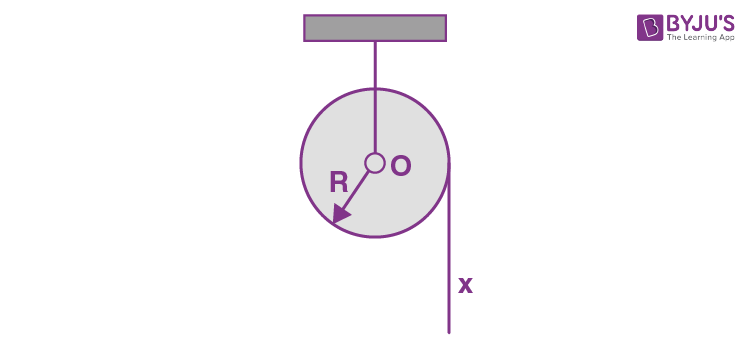Solution:

1. Let us use the equation dMz/dt = Nzrelative to the axis through O (1)

For this purpose, let us find the angular momentum of the system Mz about the given rotation axis and the corresponding torque Nz. The angular momentum is

Mz = Iω + mvR

= (m0/2 + m)R2ω

(where I = m0R2/2 and v = ωR (no cord slipping)

So dMz/dt = (MR2/2 + mR2z (2)

The downward pull of gravity on the overhanging part is the only external force, which exerts a torque about the z axis, passing through O and is given by,

Nz =(m/l)xgR

Hence from the equation dMz/dt = Nz

(MR2/2 + mR2z= (m/l)xgR

Thus, βz= 2mgx/lR(M+2m) > 0

Note: We may solve this problem using conservation of mechanical energy of the system (cylinder + thread) in the uniform field of gravity.

19. A uniform sphere of mass m and radius R rolls without slipping down an inclined plane set at an angle α to the horizontal.

Find:

1. (a) the magnitudes of the friction coefficient at which slipping is absent;

2. (b) the kinetic energy of the sphere t seconds after the beginning of motion.

Solution:

1. (a) Let us indicate the forces acting on the sphere and their points of application. Choose positive direction of x and ϕ (rotation angle) along the incline in downward direction and in the sense of $\vec{\omega }$ (for unidirectional rotation) respectively. Now from equations of dynamics o f rigid body

i.e. Fx = mwcx and Ncz = Icβz we get:

mg sin α - fr = mw (1)

And frR = ⅖ mR2β (2)

But fr ≤ kmg cos α (3)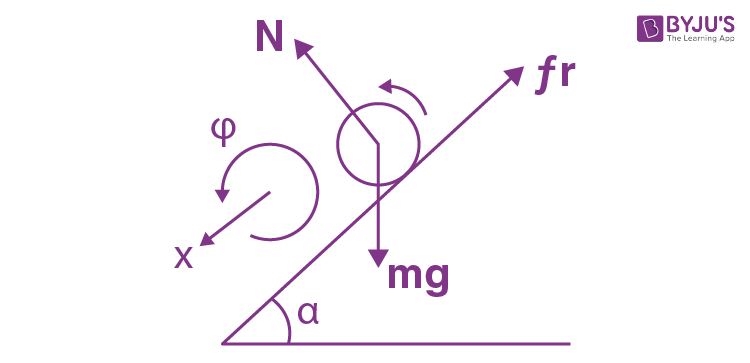In addition, the absence of slipping provides the kinematical relationship between the accelerations : w = βR (4)

The simultaneous solution of all the four equations yields:

k cos α ≥ 2/7 sin α

Or k ≥ 2/7 tan α

(b) solving Eqs. (1) and (2) [of part (a)], we get:

wc = (5/7)g sin α

As the sphere starts at t = 0 along positive x axis, for pure rolling

vc(t) = wct = (5/7)g sin αt (5)

Hence the sought kinetic energy

T = ½ mvc2 + ½ (⅖)mR2ω2 = (7/10)mvc2 (as ω = vc/R)

= (7/10)m(5g sin αt/7)2

= (5/14)mg2 sin2α t2

20. A uniform cylinder of mass m = 8.0 kg and radius R = 1.3 cm (Fig. 1.60) starts descending at a moment t = 0 due to gravity. Neglecting the mass of the thread, find:

1. (a) the tension of each thread and the angular acceleration of the cylinder;

2. (b) the time dependence of the instantaneous power developed by the gravitational force.

Solution:

1. (a) Let us indicate the forces and their points of application fox the cylinder. Choosing the positive direction for x and ϕ as shown in the figure, we write the equation of motion of the cylinder axis and the equation of moments in the C.M. frame relative to that axis

i.e. from equation Fx = mwc and Nz = Icβz

mg - 2T = mwc

2TR = mR2β/2

As there is no slipping of thread on the cylinder

wc = βR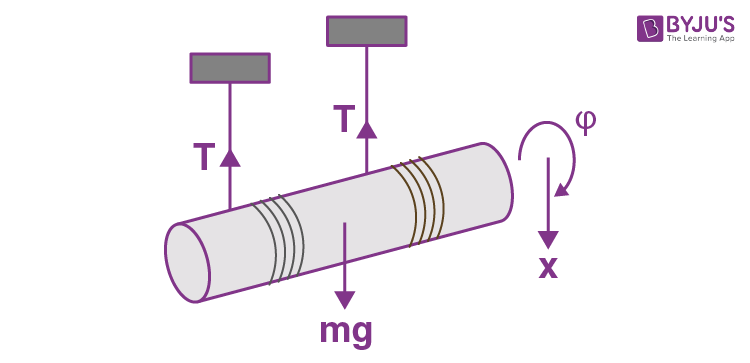From these three equations

T = mg/6 = 13 N

β = 2g/5R = 5×102 rad/s2

(b) we have β = 2g/3R

So wc = 2g/3 > 0 or in vector form $\vec{w }_{c}=\frac{2}{3}\vec{g}$

P = $\vec{F}.\vec{v}=\vec{F}.(\vec{w}_{c}t)$

= $m\vec{g}.(\frac{2}{3}\vec{g}t)$

= ⅔ mg2 t